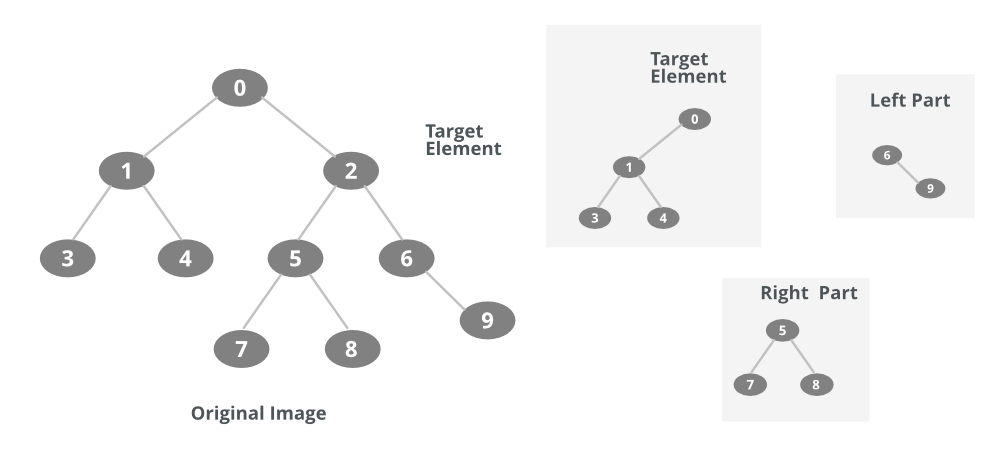# Java Program to Partition a Tree from a Given Element using DFS

• Difficulty Level : Hard
• Last Updated : 29 Oct, 2021

Depth First Traversal (or Search) for a graph is similar to Depth First Traversal of a tree. The only catch here is, unlike trees, graphs may contain cycles, a node may be visited twice. To avoid processing a node more than once, use a boolean visited array. DFS is a traversal method used to find the spanning tree out of a given graph or a tree.

To partition a tree from a given element means that we have to find different strongly connected trees generated by removing that element from the tree. So we will be using DFS to find different components of a partitioned tree which is formed after removing the given element. Hence, the DFS algorithm using java is a prerequisite.

Procedure: The partitioning of a tree from any element results in the following three cases:

Case 1: If that element has a parent and one left subtree and one right subtree, then there will be different spanning trees as follows:

• The first spanning tree will be that which is formed by doing DFS on the parent node of that particular element node.
• The second would result from the left subtree since all the nodes of a left subtree will get separated from the nodes of the parent and will result in a separate spanning tree.
• The third will be the right subtree as same as in the above case.

Case 2: If the node has only a parent node, then there will be only one partition (spanning tree) possible i.e. all nodes in that tree except that node.

Case 3: If the node has no parent and only child are present (root element), then if there are two children then two else one spanning tree is possible.

Case 4: If the node has only one child and a parent then two spanning trees are possible.The following figure displays and clears all the cases.

Hence, we have to write a partition function that takes the input element and the adjacency list of the tree and then calls the DFS method after deciding the cases as stated above. Then the DFS function returns the spanning tree as generated by the partition. Hence, the Java Program is given below which partitions the tree from the given element.

Example

## Java

 `// Java Program to Partition a Tree from a Given Element using DFS`   `// Importing required libraries` `import` `java.lang.*;` `import` `java.io.*;` `import` `java.util.*;`   `// Main class` `public` `class` `Main {`   `    ``public` `static` `int` `V;` `    ``// Adjacency List Representation` `    ``public` `static` `LinkedList adj[];`   `    ``// Constructor` `    ``@SuppressWarnings``(``"unchecked"``) Main(``int` `v) {` `        ``V = v;` `        ``adj = ``new` `LinkedList[v];` `        ``for` `(``int` `i = ``0``; i < v; ++i)` `            ``adj[i] = ``new` `LinkedList();` `    ``}`   `    ``// Method 1` `    ``// To add an edge into the graph` `    ``static` `void` `addEdge(``int` `v, ``int` `w) {` `        ``adj[v].add(w); ``// Add w to v's list.` `    ``}`   `    ``// Method 2` `    ``// DFS function` `    ``static` `void` `DFSUtil(``int` `v, ``boolean` `visited[]) {` `        ``visited[v] = ``true``;` `        ``System.out.print(v + ``" "``);` `        ``Iterator i = adj[v].listIterator();` `        ``while` `(i.hasNext()) {` `            ``int` `n = i.next();` `            ``if` `(!visited[n])` `                ``DFSUtil(n, visited);` `        ``}` `    ``}`   `    ``// Method 3` `    ``// To Partition the tree as it calls DFS` `    ``// for parent, Right and Left trees.` `    ``static` `void` `Partition(``int` `v) {`   `        ``Iterator i = adj[v].listIterator();` `        ``int` `k = ``1``;` `        ``boolean` `visited[] = ``new` `boolean``[V];` `        ``// Flag variable` `        ``visited[v] = ``true``;`   `        ``// The current element is separated from partition` `        ``while` `(i.hasNext()) {`   `            ``// DFS for all the partitions like parent ,left and right.` `            ``System.out.println();` `            ``System.out.println(k + ``"Tree"``);`   `            ``++k;`   `            ``int` `n = i.next();` `            ``DFSUtil(n, visited);` `        ``}` `    ``}`   `    ``// Method 4` `    ``// main driver method` `    ``public` `static` `void` `main(String args[]) {`   `        ``// Creating an object of class` `        ``// in main() method` `        ``Main g = ``new` `Main(``10``);`   `        ``// Sample illustration of a tree` `        ``// as how it will look like`   `        ``/*  0` `           ``/  \` `          ``1    2 -> Target Node.` `         ``/ \  / \` `        ``3  4 5   6`   `            ``/ \   \` `           ``7   8   9` `        ``*/`   `        ``// Adding edges passing arguments` `        ``// as per above generated tree` `        ``g.addEdge(``0``, ``1``); g.addEdge(``1``, ``0``);` `        ``g.addEdge(``0``, ``2``); g.addEdge(``2``, ``0``);` `        ``g.addEdge(``1``, ``3``); g.addEdge(``3``, ``1``);` `        ``g.addEdge(``1``, ``4``); g.addEdge(``4``, ``1``);` `        ``g.addEdge(``2``, ``5``); g.addEdge(``5``, ``2``);` `        ``g.addEdge(``2``, ``6``); g.addEdge(``6``, ``2``);` `        ``g.addEdge(``5``, ``7``); g.addEdge(``7``, ``5``);` `        ``g.addEdge(``5``, ``8``); g.addEdge(``8``, ``5``);` `        ``g.addEdge(``6``, ``9``); g.addEdge(``9``, ``6``);`   `        ``// Calling the Method3 to` `        ``// partition the tree` `        ``Partition(``2``);` `    ``}` `}`

Output

```1Tree
0 1 3 4
2Tree
5 7 8
3Tree
6 9 ```

My Personal Notes arrow_drop_up
Recommended Articles
Page :